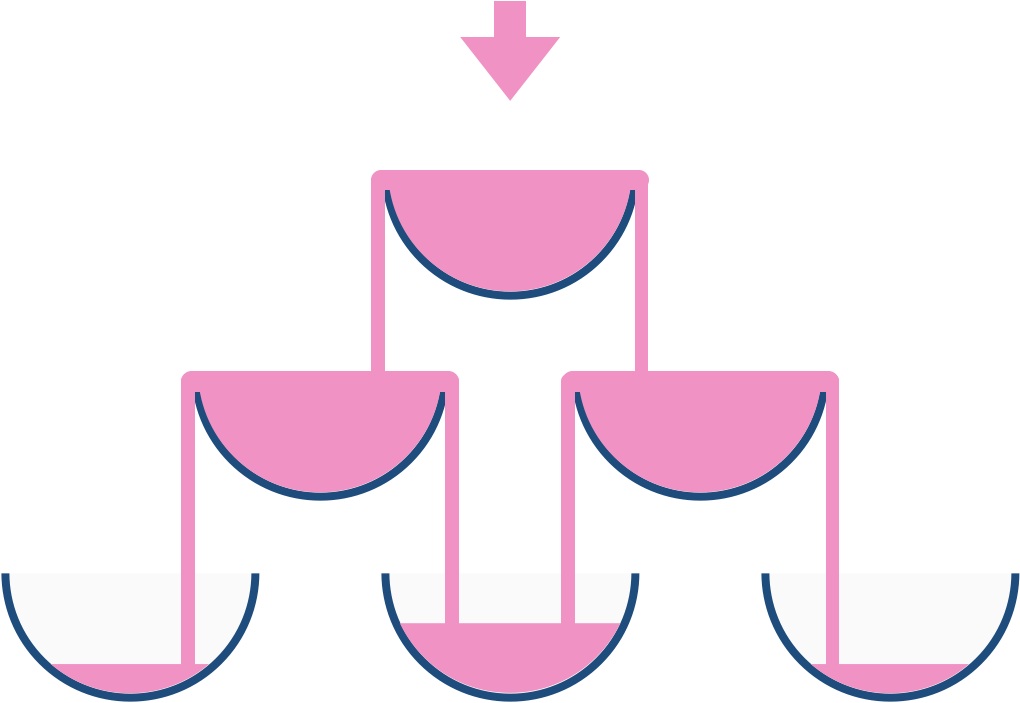# LeetCode: 799. 香槟塔¶

## 1、题目描述¶输入: poured(倾倒香槟总杯数) = 1, query_glass(杯子的位置数) = 1, query_row(行数) = 1



输入: poured(倾倒香槟总杯数) = 2, query_glass(杯子的位置数) = 1, query_row(行数) = 1



• poured 的范围[0, 10 ^ 9]。
• query_glass 和query_row 的范围 [0, 99]。

## 2、解题思路¶

• dfs+记忆化

• 判断当前杯子流过的香槟有多少，就需知道上面流经上放的杯子的香槟的量，并且减去上方杯子本身的量

from functools import lru_cache

class Solution:
def champagneTower(self, poured: int, query_row: int, query_glass: int) -> float:

@lru_cache()
def dfs(i, j):
if i == 0 and j == 0:
return poured
if j < 0 or j > i:
return 0

left = max(dfs(i - 1, j - 1) - 1, 0) / 2
right = max(dfs(i - 1, j) - 1, 0) / 2
return left + right

ans = dfs(query_row, query_glass)

return min(1.0, ans)